Thevenin and Norton Equivalent Circuits
More tools for reducing circuits.

The Thevenin and Norton Equivalent Circuits:

### Consider the following two circuits:

 This is a Thevenin subcircuit If we apply KVL to this circuit we get the following equation: -VT + IRT + Vx = 0 Vx = VT - IRTThis is a Norton subcircuit If we apply KCL to this circuit we get the following equation: IN = Vx/RN + I Vx/RN = IN - I Vx = INRN - IRN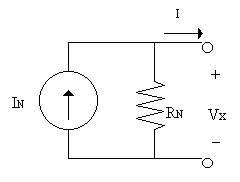Recalling from an early lecture, we stated that if two cicuits yield the same mathematical equation then the two circuits are electrically equivalent as well. How can we make these two equations the same? These two circuits (& equations) are the same if the following is true: RT = RN VT = INRN

Since the Thevenin and Norton subcircutis are equivalent if RT = RN and VT = INRN
then one subcircuit can be exchanged for the other in any circuit problem. The process of exchanging one for the other is called

Source Transformations

By performing source transformations, it can be much simpler to reduce a circuit.

## Study Problem

After clicking on the following link enter 3-1 for the problem and 1 for the step:
Study Problem 3-1

Top of Page

Finding the Thevenin and Norton Equivalent Circuits:

In the previous section we show how to do Source Transformations to reduce a circuit.
In this section we will show another method for obtaining either the Thevenin or Norton
subcircuits from a complex circuit.

This method involves looking at the voltage and current relationships at a pair of terminals
to determine what the equivalent circuit is.

### Consider the following two subcircuits:

This is a portion of the circuit you saw in problem 3-1.

We know from 3-1 that this subcircuit reduces to the subcircuit shown at the right.
In 3-1 we used source transformations to find the equivalent circuit, however now we will look at the current and voltage values at the output terminals.

If the two circuits are equivalent then the voltage and current measured at the terminals should be identical.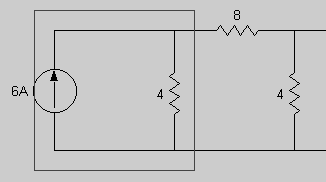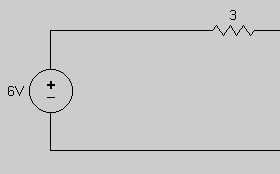Calculating the Open-Circuit Voltage

Both circuits above should have the same open circuit voltage as measured from the two terminals on the right:

We will first calculate Vopen-circuit or Voc for the more complex circuit:

Using current division: I8 = 6A[4/(4+12)] = 6A(1/4) = 3/2 A

Note that the 4 and 12 Ohm resistors are in series since there is no current flowing to the output terminal.

Voc = I8*4 Ohms = 3/2 A * 4 Ohms = 6 Volts

Both the complex and the reduced circuits should have the same open-circuit voltage as measured
from the two output terminals on the right: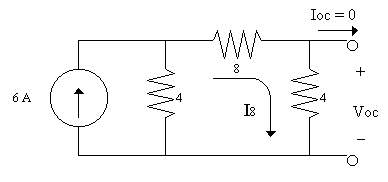Calculating the Short-Circuit Current

Now let's find the current across the output terminals when they are shorted by a wire.
We will call this current Ishort-circuit or Isc because it is the current which flows from one output terminal to the other when the two terminals are shorted together.
'Shorted' means the two terminals are connected by a wire.
Isc = I8 because there is no current in the 4 Ohm resistor.
Since the rightmost 4 Ohm resistor is shorted by a wire the voltage across the 4 Ohm resistor is zero.
If V=IR and Vsc=0 then I in the 4 Ohm resistor has to be zero.

The 4 Ohm resistor has no effect on the circuit because no current flows through it.
You could remove the rightmost 4 Ohm resistor if you wanted to for this step.

Therefore applying Current Division:
I8 = Isc = 6A[4/(4+8)] = 6A(1/3) = 2A

Both the complex and the reduced circuits should have the same short-circuit current as measured
from the two output terminals on the right:Now we calculate the Open-Circuit Voltage for the reduced Thevenin circuit.

I=0 because the circuit is open. Current has no where to flow.

KVL in the loop gives:
-6V + 3I + Voc = 0
Plugging in I=0:
Voc = 6 Volts

This is the same open-circuit voltage found with the more complex circuit.

NOTE: Voc = VTNow we calculate the Short-Circuit Current for the reduced Thevenin circuit.

Here the loop current is equal to Isc and Vsc = 0.
Applying KVL to this circuit:

-6V + 3Isc + 0 = 0
6V = 3Isc
Isc = 6V/3Ohms = 2A

or Isc = VT/RT

This is the same short-circuit current found with the more complex circuit.

Since IN = VT/RT, then: Isc = IN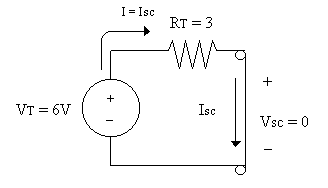Summary:
To find the Thevenin equivalent circuit for a linear circuit open up the output terminals
and calculate Voc.

### VT = Voc

To find RT you need Isc because Isc = IN
Recall that RT = VT/IN = Voc/Isc

To find Isc, short out the output terminals with a wire and calculate
the current that flows from one terminal to the other.

### RT = Voc/Isc

Getting the Norton Equivalent circuit is done the same way since IN = Isc
and RN = RT.

### It is important to note that a linear circuit can be reduced to a Thevenin or Norton Equivalent, however a circuit which is not linear can not be reduced to a Thevenin or Norton subcircuit.The linear complex circuit must yield an equation which takes the form of F(I) at the output terminals. If it does not, then the circuit can not be replaced with a two element Thevenin or Norton Equivalent.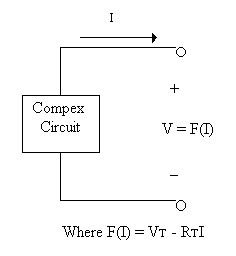Top of Page

Practice reducing a circuit to its Thevenin or Norton Equivalent

In the previous section we see how to find the Thevenin or Norton Equivalent Circuit for a complex
linear circuit. In this section we will go through examples to reinforce these concepts.

However, first let's look at how to find RT or RN directly with out having to find both Voc and Isc.

## Consider the following examples:

Finding RT or RN directly:

To find the Thevenin/Norton Equivalent resistance you must set all independent sources to zero.
Recall F(I) = VT - RTI.
By setting all sources to zero in a subcircuit, this sets VT in this equation to zero. If the terminals are left open that sets I to zero as well.

#### Thus only RT is left.

Practically how is this done:
First kill all independent sources (this means set voltage sources to zero volts and set current sources to zero amps). Then open up the output terminals. Calculate the resistance from one terminal to the other.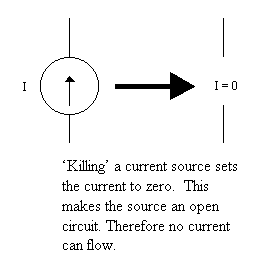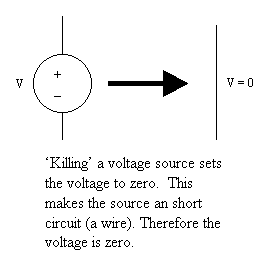Finding RT in the first example: We need to find RT as seem between Terminal-1 and Terminal-2.
The first step is to kill all independent sources. This is like unplugging
the circuit and letting voltage sources be wires and current sources be open
circuits. This "dead" circuit is shown on the right.

Next we need to find the resistance of this "dead" circuit.
Note that the 2 Ohm is in parallel with some open ciruits.
Note that 2 Ohms in parallel with infinity = 2 Ohms.
So the three resistors are in series.
RT = (5 + 2 + 8)Ohms = 15 Ohms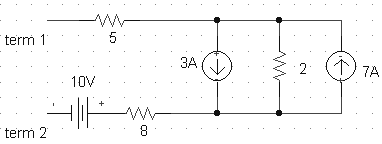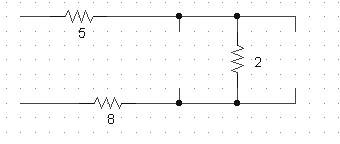Finding RT - second example:
In this example the terminals are on the right-hand side. We still need to find RT as seem between Terminal-1 and Terminal-2. Again the first step is to kill all independent sources. The dead circuit is shown on the right.

Next we need to find the resistance of this "dead" circuit.
Note that the 10 Ohms is in series with an 'open' (effectively infinite resistance). This makes the 10 Ohm branch infinite resistance, so we can ignore this branch.

We have two four Ohms resistors in parallel with each other. 4//4 = 2 (Note: '//' means 'in parallel')
RT = (8 + 4//4)Ohms = (8 + 2)Ohms = 10 Ohms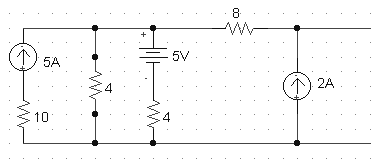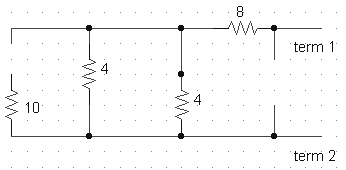Finding RT in the first example using a different method:
Another way to find RT or RN is by exciting the dead circuit with an external independent source.
The first step is to again 'kill' all independent sources.
The second step is to place an independent source across the terminals, any current or voltage source will do.
The third step involves finding the current and voltage at the terminals.
Then RT is = Vin/Iin

Finding Iin: First we do KVL -
-1V + 5Iin + 2Iin + 8Iin = 0
1V = 15Iin
Iin = 1/15 Amps
RT = Vin/Iin = 1 Volt/(1/15 Amps) = 15 Ohms
This is the same answer found in the first example.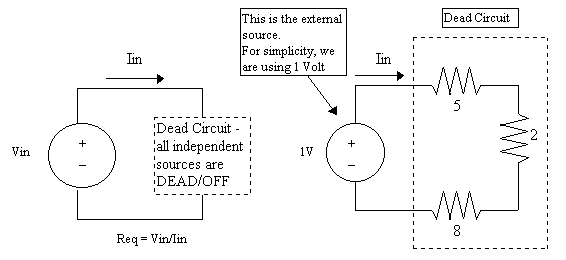## Study Problems

After clicking on the following link enter 3-2 for the problem and 1 for the step:
Study Problem 3-2
After clicking on the following link enter 3-3 for the problem and 1 for the step:
Study Problem 3-3
After clicking on the following link enter 3-4 for the problem and 1 for the step:
Study Problem 3-4

Top of Page

Practical Sources

For analysis purposes we assume that voltage and current sources are perfect.

What is a perfect voltage source?
A voltage source which is the specified value no matter what.
In other words... if the specified voltage is 10V then the voltage across the source is 10 Volts always
no matter what is connected to the source.

What is a perfect current source?
A current source which is the specified value no matter what.
In other words... if the specified current is 10A then the current through the source is 10 Amps always
no matter what is connected to the source.

Perfect Sources exist on paper only. In the lab we will find out that sources
are not perfect and that they ARE affected by what is connected to them.

For example, if you short out a perfect voltage source and apply V=IR then you would calculate infinite current.
No power supply is going to output infinite current.
In reality the voltage across the source will drop.

In another example, if you place a resistor in parallel with a perfect current source, then as that resistor approaches infinity
then the power delivered by the source approaches infinity. This is impossible in the real world.
In reality the current from the source will drop.

Sources which behave like real world sources are called Practical Sources:
 The Practical Voltage Source: A real world voltage source (i.e. practical voltage source) is modeled by what you see enclosed in the box. Note that a practical voltage source has some small but measureable internal resistance, Rint. If the load resistor RL approaches zero, the current from the source can not exceed VS/Rint. Thus current through the load does not approach infinity. With a perfect voltage source the current through the load can theoretically approach infinty.The Practical Current Source: A real world current source (i.e. practical current source) is modeled by what you see enclosed in the box. Note that a practical current source has some small but measureable internal resistance, Rint. If the load resistor RL approaches infinity (an open circuit), the current from the source into the load will approach zero. Voltage across the practical current source can not exceed IS*Rint. Thus voltage across the load does not approach infinity. With a perfect current source the voltage across the load can theoretically approach infinty.Note that the current source and the voltage source are equivalent circuits. The voltage source is the Thevenin Circuit and the current source is the Norton Circuit. So if we never see a current source in the lab, why do we care about the current source on paper? The current source is a mathematical model which is can be used to simplify circuit analysis. However it may never be built in the real world.

Top of Page

Back To Index